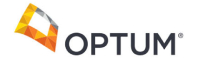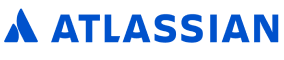New update is available. Click here to update.

# Median of two sorted arrays

Contributed by
Prashant Thakur
Hard0/120
25 mins
65 %84 upvotes+27 more

## Problem Statement

#### The expected time complexity is O(min(logn, logm)), where 'n' and 'm' are the sizes of arrays 'a' and 'b', respectively, and the expected space complexity is O(1).

##### Example:
``````Input: 'a' = [2, 4, 6] and 'b' = [1, 3, 5]

Output: 3.5

Explanation: The array after merging 'a' and 'b' will be { 1, 2, 3, 4, 5, 6 }. Here two medians are 3 and 4. So the median will be the average of 3 and 4, which is 3.5.
``````
Detailed explanation ( Input/output format, Notes, Images )
##### Sample Input 1:
``````3 3
2 4 6
1 3 5
``````
##### Sample Output 1:
``````3.5
``````
##### Explanation of Sample Input 1 :
``````The array after merging 'a' and 'b' will be { 1, 2, 3, 4, 5, 6 }.
Here two medians are 3 and 4. So the median will be the average of 3 and 4, which is 3.5.
``````
##### Sample Input 2:
``````3 2
2 4 6
1 3
``````
##### Sample Output 2:
``````3
``````
##### Explanation of Sample Input 2 :
``````The array after merging 'a' and 'b' will be { 1, 2, 3, 4, 6 }.
The median is 3.
``````
##### Sample Input 3:
``````3 3
1 2 2
2 4 4
``````
##### Sample Output 3:
``````2.0
``````
##### Explanation of Sample Input 3 :
``````The array after merging 'a' and 'b' will be { 1, 2, 2, 2, 4, 4 }.
Here two medians are 2 and 2. So the median will be the average of 2 and 2, which is 2.
``````
##### Constraints:
``````1 <= 'n' <= 10 ^ 6
1 <= 'm' <= 10 ^ 6
1 <= 'a[i]' <= 10 ^ 9
1 <= 'b[i]' <= 10 ^ 9

Time limit: 1 sec.
``````AutocompleteConsole